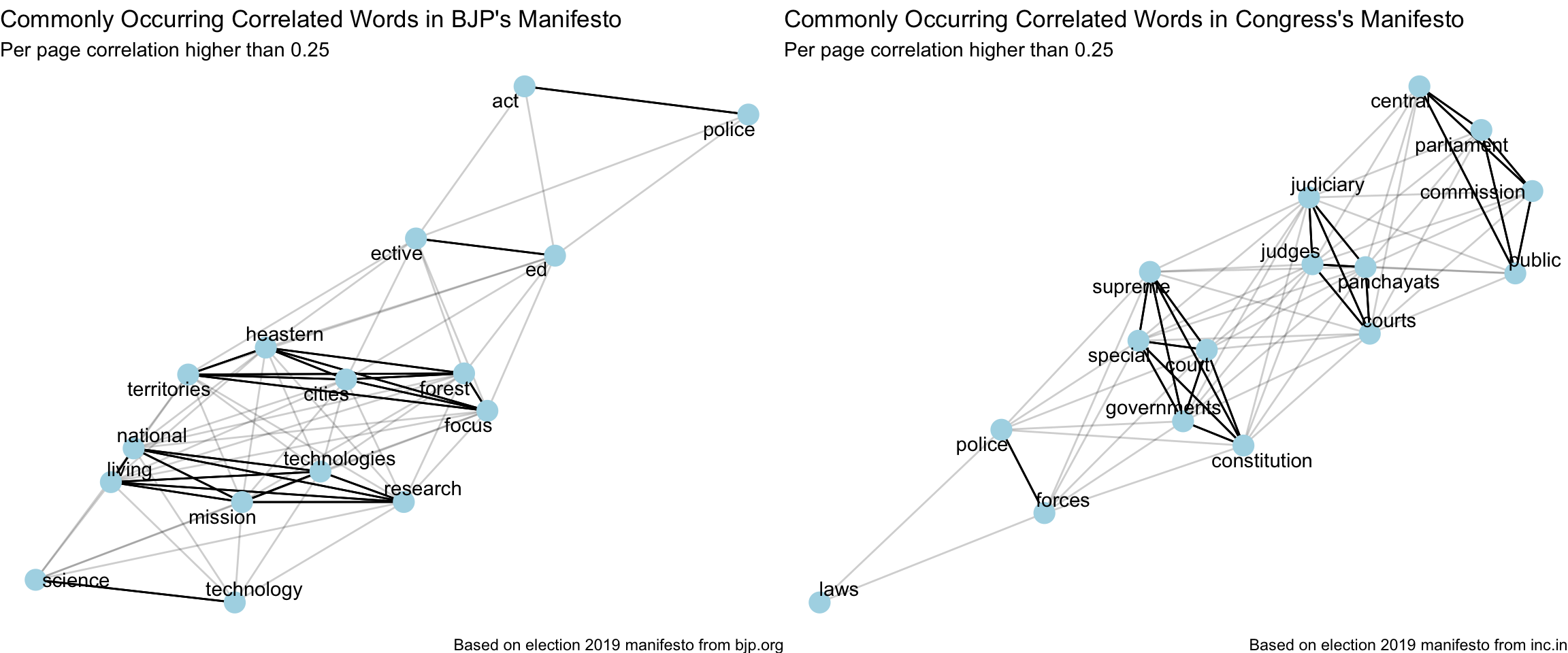# Introduction

With India’s 2019 General Elections around the corner, I thought it’d be a good idea to analyse the election manifestos of its 2 biggest political parties, BJP and Congress. Let’s use text mining to understand what each party promises and prioritizes.
In this part 4, I’ll explore the Anti-corruption and Good Governance discussions in both manifestos.

# Analysis

``````rm(list = ls())
library(tidyverse)
library(pdftools)
library(tidylog)
library(hunspell)
library(tidytext)
library(ggplot2)
library(gridExtra)
library(scales)
library(reticulate)
library(widyr)
library(igraph)
library(ggraph)

theme_set(theme_light())
use_condaenv("stanford-nlp")``````

``````bjp_content <- read_csv("../data/indian_election_2019/bjp_manifesto_clean.csv")

## Anti-Corruption and Good Governance

This topic is covered congress’ manifesto from Pages 17 to 19 of the pdf and in that of bjp’s from pages 24 to 26.

``````bjp_content %>%
filter(between(page, 24, 26)) -> bjp_content

congress_content %>%
filter(between(page, 17, 19)) -> congress_content``````

## Common correlated words

``````plot_common_correlated_words <- function(df,
counts_quantile = 0.7,
correlation_threshold = 0.25,
stop_words_list = stop_words) {
set.seed(123)
df %>%
unnest_tokens(word, text) %>%
anti_join(stop_words_list) %>%
filter(n > stats::quantile(n, counts_quantile)) %>%
pairwise_cor(word, page, sort = TRUE) %>%
filter(correlation > correlation_threshold,
!str_detect(item1, "\\d"),
!str_detect(item2, "\\d")) %>%
graph_from_data_frame() %>%
ggraph(layout = "fr") +
geom_edge_link(aes(edge_alpha = correlation), show.legend = FALSE) +
geom_node_point(color = "lightblue", size = 5) +
geom_node_text(aes(label = name), repel = TRUE) +
theme_void() -> p

return(p)
}``````
``````bjp_content %>%
plot_common_correlated_words(stop_words_list = custom_stop_words,
counts_quantile = 0.75) +
labs(x = "",
y = "",
title = "Commonly Occurring Correlated Words in BJP's Manifesto",
subtitle = "Per page correlation higher than 0.25",
caption = "Based on election 2019 manifesto from bjp.org") -> p_bjp

congress_content %>%
plot_common_correlated_words(stop_words_list = custom_stop_words,
counts_quantile = 0.75) +
labs(x = "",
y = "",
title = "Commonly Occurring Correlated Words in Congress's Manifesto",
subtitle = "Per page correlation higher than 0.25",
caption = "Based on election 2019 manifesto from inc.in") -> p_congress

grid.arrange(p_bjp, p_congress, ncol = 2, widths = c(12,12))``````## Basic Search Engine

Lets build a cosine-similarity based simple search engine (instead of the basic keyword-based search that comes with the pdf document), in order to make these documents more easily searchable and gain context using most related lines in the manifestos for a given query. Using python’s scikit-learn for this.

``````from sklearn.feature_extraction.text import TfidfVectorizer, ENGLISH_STOP_WORDS
from sklearn.metrics.pairwise import linear_kernel

stopwords = ENGLISH_STOP_WORDS

vectorizer_bjp = TfidfVectorizer(analyzer='word', stop_words=stopwords, max_df=0.3, min_df=2)
vector_train_bjp = vectorizer_bjp.fit_transform(r["bjp_content\$text"])

vectorizer_congress = TfidfVectorizer(analyzer='word', stop_words=stopwords, max_df=0.3, min_df=2)
vector_train_congress = vectorizer_congress.fit_transform(r["congress_content\$text"])

def get_related_lines(query, party="bjp"):
if (party == "bjp"):
vectorizer = vectorizer_bjp
vector_train = vector_train_bjp
else:
vectorizer = vectorizer_congress
vector_train = vector_train_congress
vector_query = vectorizer.transform([query])
cosine_sim = linear_kernel(vector_query, vector_train).flatten()
return cosine_sim.argsort()[:-10:-1]``````
``get_related_lines <- py_to_r(py\$get_related_lines)``